# 【论文阅读】Distribution-Aware Coordinate Representation for Human Pose Estimation

## DARK

Posted by x-jeff on October 27, 2022

# 1.Introduction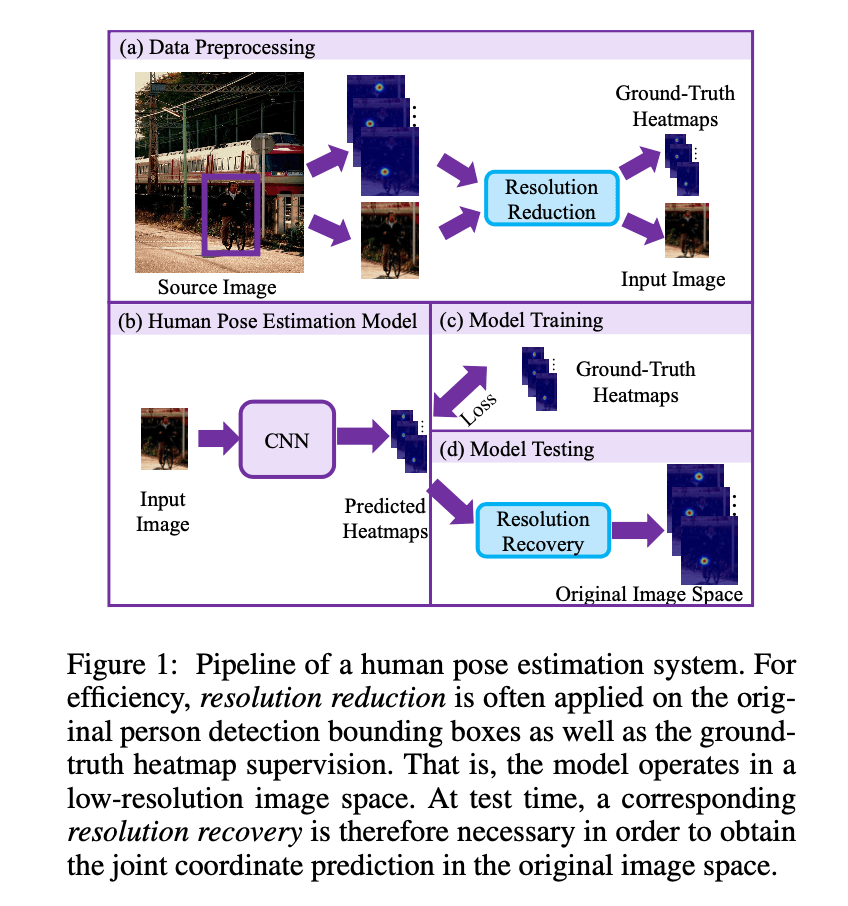Fig1展示了人体姿态估计系统的pipeline。为了提高效率，通常会对裁剪的人物图像以及对应的ground-truth heatmap进行下采样，即降低分辨率。因此，模型直接处理低分辨率图像。在推理阶段，关节点坐标会被恢复至原始图像分辨率（resolution recovery）。

HRNet-W32论文：Sun, K.; Xiao, B.; Liu, D.; and Wang, J. 2019. Deep high-resolution representation learning for human pose estimation. In IEEE Conference on Computer Vision and Pattern Recognition.。

# 2.Related Work

👉Coordinate regression

👉Heatmap regression

heatmap的设计巧妙的解决了上述限制。其首次提出是在论文“Tompson,J.J.; Jain,A.; LeCun,Y.; and Bregler, C. 2014. Joint training of a convolutional network and a graphical model for human pose estimation. In Advances in Neural Information Processing Systems.”中，并迅速成为最常用的坐标表示方法。目前主流的研究都聚焦于设计网络结构以更有效的训练heatmap。

# 3.Methodology

## 3.1.Coordinate Decoding

### 3.1.1.The standard coordinate decoding method

$\mathbf{p} = \mathbf{m} + 0.25 \frac{\mathbf{s}-\mathbf{m}}{\parallel \mathbf{s}-\mathbf{m} \parallel _2} \tag{1}$

$\hat{\mathbf{p}} = \lambda \mathbf{p} \tag{2}$

$\lambda$为下采样比例（resolution reduction ratio）。

👉Remarks

### 3.1.2.The proposed coordinate decoding method

$\mathcal{G} (\mathbf{x}; \mathbf{\mu}, \Sigma) = \frac{1}{(2\pi) \lvert \Sigma \rvert^{\frac{1}{2}}} exp \left( -\frac{1}{2} (\mathbf{x}-\mathbf{\mu})^T \Sigma^{-1} (\mathbf{x} - \mathbf{\mu}) \right) \tag{3}$

$\mathbf{x}$为预测的heatmap中的像素坐标，均值$\mathbf{\mu}$可理解为被预测的目标关节点的坐标。协方差矩阵$\Sigma$为对角矩阵：

$\Sigma = \begin{bmatrix} \sigma^2 & 0 \\ 0 & \sigma^2 \\ \end{bmatrix} \tag{4}$

$\sigma$为标准差，两个方向（即x方向和y方向）的标准差是一样的。

$\mathcal{P} (\mathbf{x}; \mathbf{\mu}, \Sigma) = \ln (\mathcal{G}) = -\ln (2\pi) - \frac{1}{2} \ln (\lvert \Sigma \rvert) - \frac{1}{2} (\mathbf{x} - \mathbf{\mu})^T \Sigma^{-1} (\mathbf{x} - \mathbf{\mu}) \tag{5}$

$\mathcal{D}'(\mathbf{x}) \big| _{\mathbf{x}=\mathbf{\mu}} = \frac{\partial \mathcal{P}^T}{\partial \mathbf{x}} \big| _{\mathbf{x}=\mathbf{\mu}} = -\Sigma ^{-1} (\mathbf{x} - \mathbf{\mu}) \big| _{\mathbf{x}=\mathbf{\mu}} = 0 \tag{6}$

$\mathcal{P} (\mathbf{\mu}) = \mathcal{P}(\mathbf{m}) + \mathcal{D}' (\mathbf{m}) (\mathbf{\mu}-\mathbf{m})+\frac{1}{2} (\mathbf{\mu}-\mathbf{m})^T \mathcal{D}''(\mathbf{m}) (\mathbf{\mu}-\mathbf{m}) \tag{7}$

$\mathcal{D}'' (\mathbf{m}) = \mathcal{D}'' (\mathbf{x}) \big| _{\mathbf{x} = \mathbf{m}} = -\Sigma^{-1} \tag{8}$

$\mathbf{\mu} = \mathbf{m} - (\mathcal{D}''(\mathbf{m}))^{-1} \mathcal{D}' (\mathbf{x}) \tag{9}$

👉Remarks

### 3.1.3.Heatmap distribution modulation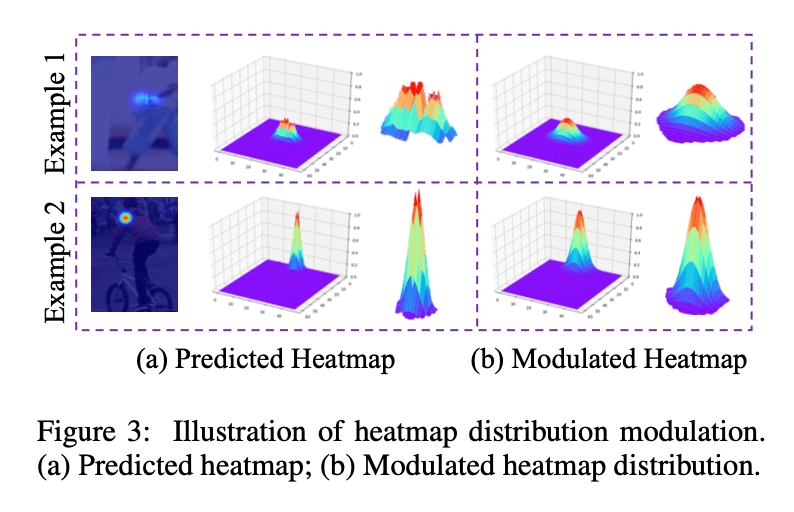Fig3中，(a)为预测得到的heatmap分布，(b)为修改后的heatmap分布。

$\mathbf{h}' = K \circledast \mathbf{h} \tag{10}$

$\circledast$表示卷积操作。

$\mathbf{h}' = \frac{\mathbf{h}' - \min (\mathbf{h}')}{\max (\mathbf{h}') - \min (\mathbf{h}')} * \max (\mathbf{h}) \tag{11}$

### 3.1.4.Summary

1. Heatmap distribution modulation（见式(10),(11)）
2. 通过泰勒展开，基于分布感知，在亚像素水平上实现关节点的精准定位（见式(3)-(9)）
3. 将关节点坐标恢复至原始分辨率下（见式(2)）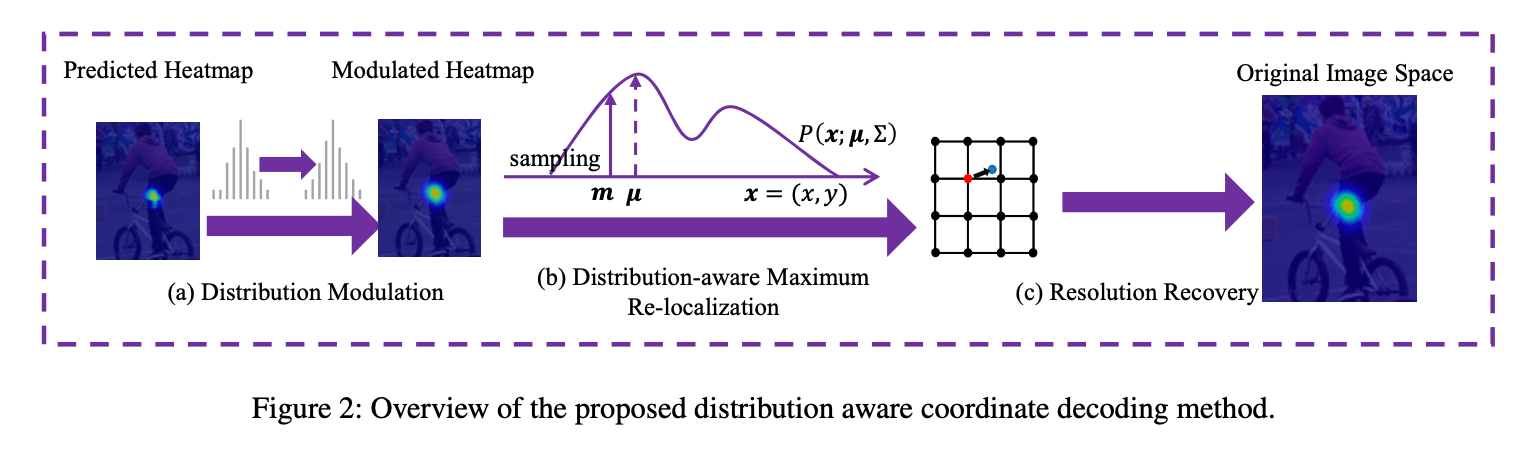## 3.2.Coordinate Encoding

$\mathbf{g}' = (u',v') = \frac{\mathbf{g}}{\lambda} = (\frac{u}{\lambda},\frac{v}{\lambda}) \tag{12}$

$\lambda$为下采样比例。

$\mathbf{g}'' = (u'',v'') = \text{quantise} (\mathbf{g}') = \text{quantise} (\frac{u}{\lambda},\frac{v}{\lambda}) \tag{13}$

$\mathcal{G} (x,y;\mathbf{g}'')=\frac{1}{2\pi \sigma^2} exp \left( -\frac{(x-\mu '')^2 + (y-v'')^2}{2\sigma^2} \right) \tag{14}$

$(x,y)$为heatmap中某一像素点的坐标。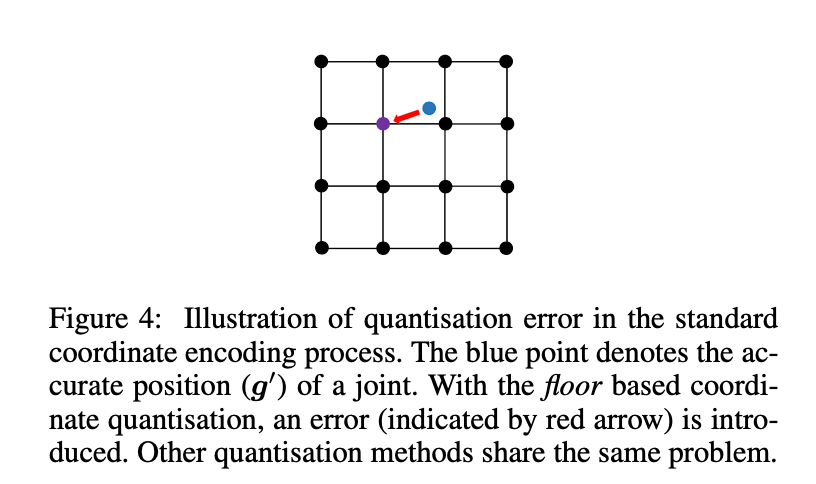Fig4阐述了标准坐标编码过程中的量化误差。图中蓝色的点表示准确的关节点坐标（即$\mathbf{g}’$）。如果采用向下取整的策略，则红色的箭头就代表了量化误差。其他的量化方法也存在同样的问题。

# 4.Experiments

👉Datasets

• COCO keypoint数据集包含不同的人体姿态、各种背景环境、不同大小的人和不同的遮挡模式。整个目标包括人物实例（person instance）和关节点位置。一共包含200,000张图像，250,000个人物实例。每个人物实例标记17个关节点。训练集和验证集的标注是公开的。在评估时，使用常用的train2017/val2017/test-dev2017的数据集划分方式。
• MPII人体姿态数据集包含40k个人物实例，每个人物实例标记16个关节点。训练集，验证集，测试集的划分遵循论文“Tompson,J.J.; Jain,A.; LeCun,Y.; and Bregler, C. 2014. Joint training of a convolutional network and a graphical model for human pose estimation. In Advances in Neural Information Processing Systems.”。

👉Evaluation metrics

👉Implementation details

Simple-Baseline原文：Xiao, B.; Wu, H.; and Wei, Y. 2018. Simple baselines for human pose estimation and tracking. In European Conference on Computer Vision.。

Hourglass原文：Newell,A.; Yang,K.; and Deng,J. 2016. Stacked hourglass networks for human pose estimation. In European Conference on Computer Vision.。

## 4.1.Evaluating Coordinate Representation

### 4.1.1.Coordinate decoding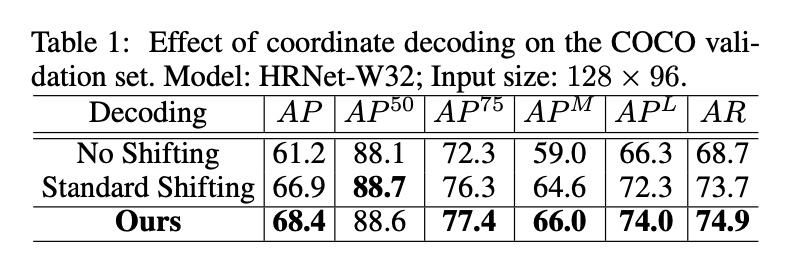1. 相比无shifting操作，标准解码方法将AP提升了5.7%，效果非常好。据我们所知，这是文献中首次报道的有效性分析，因为这一问题在很大程度上被以前的研究所忽视。这揭示了先前未发现的坐标解码过程对人体姿态估计的重要性。
2. 尽管标准解码方法将性能提升了很多，但是我们的方法将AP在此基础上又提高了1.5%。这1.5%中有0.3%的提升来自distribution modulation，见表2。这验证了我们提出的解码方法的优越性。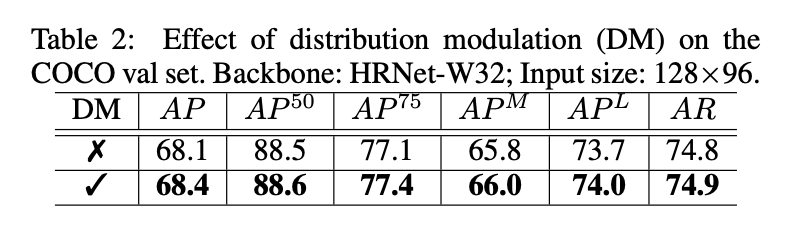### 4.1.2.Coordinate encoding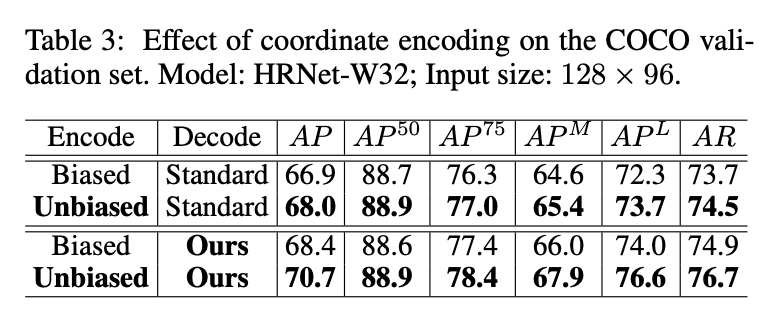### 4.1.3.Input resolution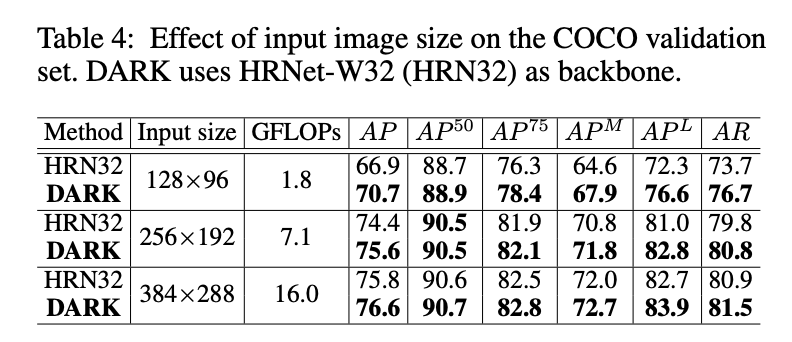1. 正如预期的那样，随着输入图像尺寸的减小，模型性能不断下降，但是其推理成本也在明显下降。
2. 在DARK的加持下，可以有效减轻模型性能的损失，特别是在输入分辨率非常小的时候。这有助于在低资源设备上部署人体姿态估计模型。

### 4.1.4.Generality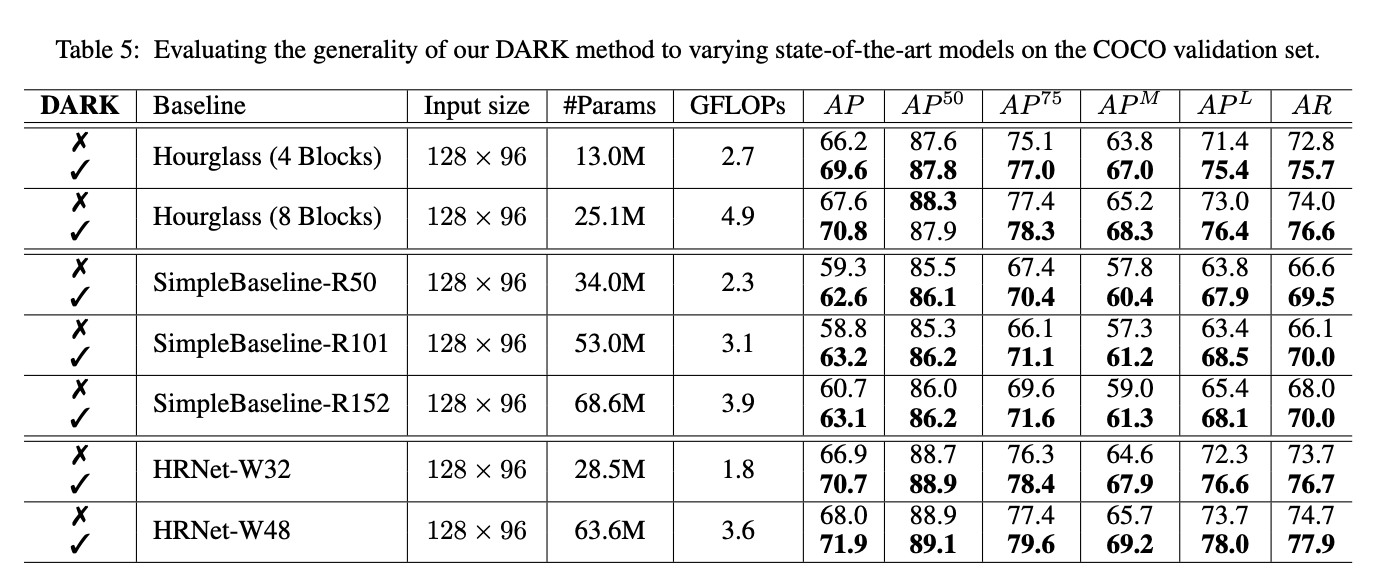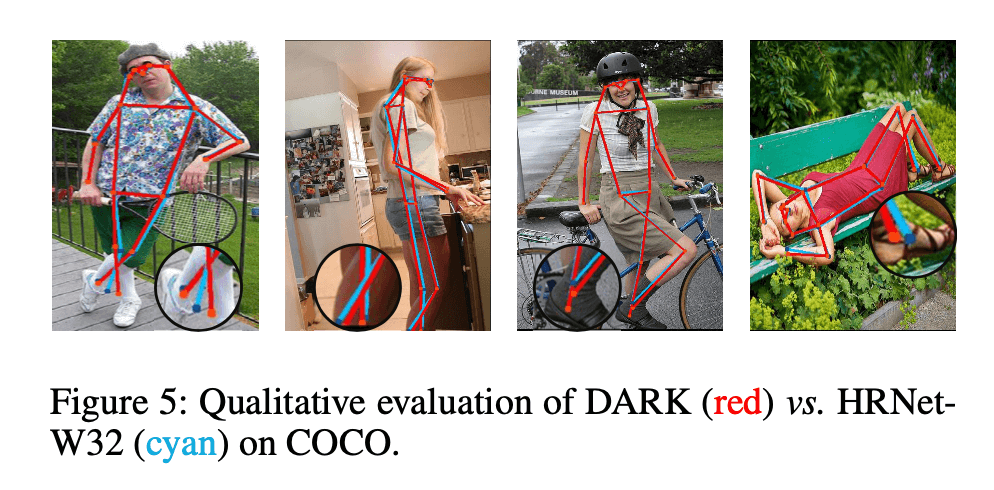## 4.2.Comparison to the State-of-the-Art Methods

### 4.2.1.Evaluation on COCO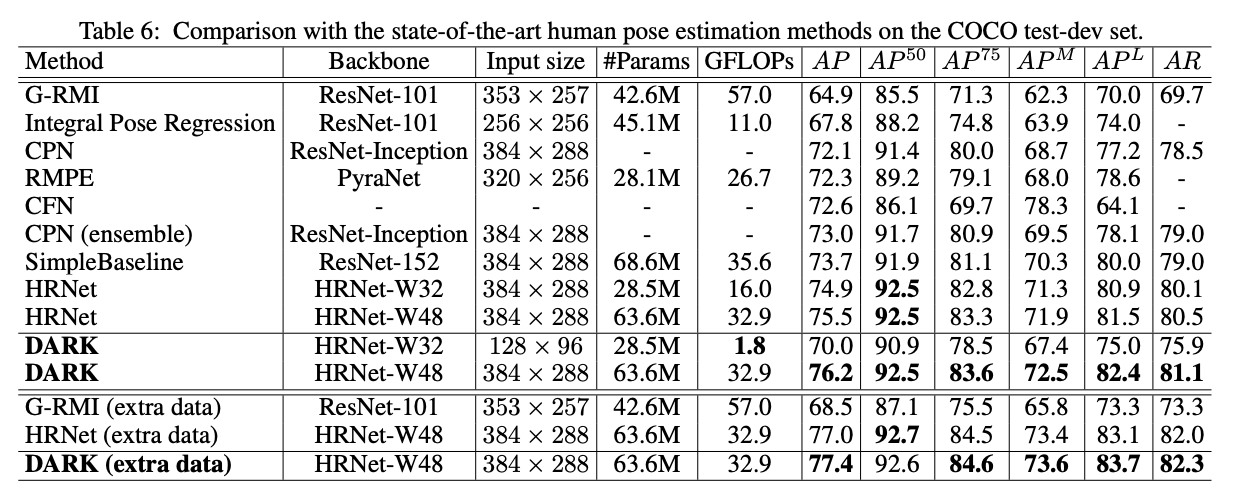1. 基于HRNet-W48且输入大小为$384 \times 288$的DARK模型准确率是最高的，并且计算成本只增加了一点点。尤其是和最强劲的对手（HRNet-W48，输入大小也为$384 \times 288$）比较时，DARK将AP提升了0.7%（76.2-75.5）。当和最有效率的模型（Integral Pose Regression，即GFLOPs最低）比较时，DARK（基于HRNet-W32）将AP提升了2.2%（70.0-67.8），但计算成本只有原来的16.4%（1.8/11.0 GFLOPs）。这些都表明了DARK在准确性和效率方面优于现有模型。

G-RMI原文：Papandreou, G.; Zhu, T.; Kanazawa, N.; Toshev, A.; Tompson, J.; Bregler, C.; and Murphy, K. 2017. Towards accurate multi-person pose estimation in the wild. In IEEE Conference on Computer Vision and Pattern Recognition, 4903– 4911.。

Integral Pose Regression原文：Sun, X.; Xiao, B.; Wei, F.; Liang, S.; and Wei, Y. 2018. Integral human pose regression. In European Conference on Computer Vision.。

CPN原文：Chen,Y.; Wang,Z.; Peng,Y.; Zhang,Z.; Yu,G.; and Sun, J. 2018. Cascaded pyramid network for multi-person pose estimation. In IEEE Conference on Computer Vision and Pattern Recognition.。

RMPE原文：Fang, H.-S.; Xie, S.; Tai, Y.-W.; and Lu, C. 2017. Rmpe: Regional multi-person pose estimation. In IEEE Conference on Computer Vision and Pattern Recognition, 2334–2343.。

### 4.2.2.Evaluation on MPII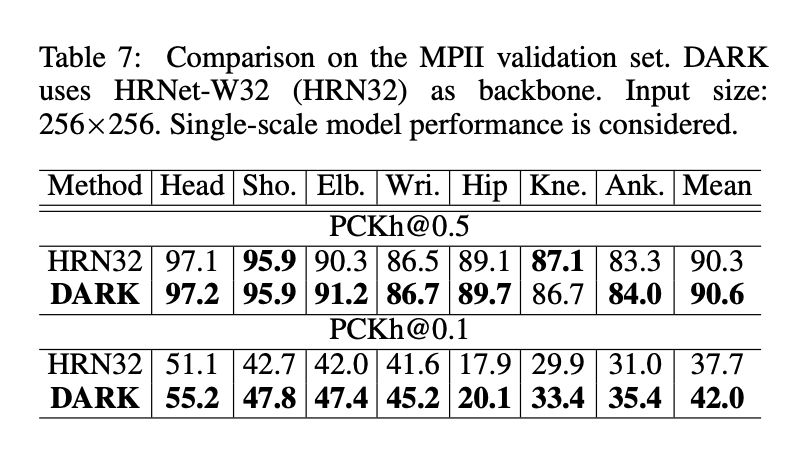# 6.原文链接